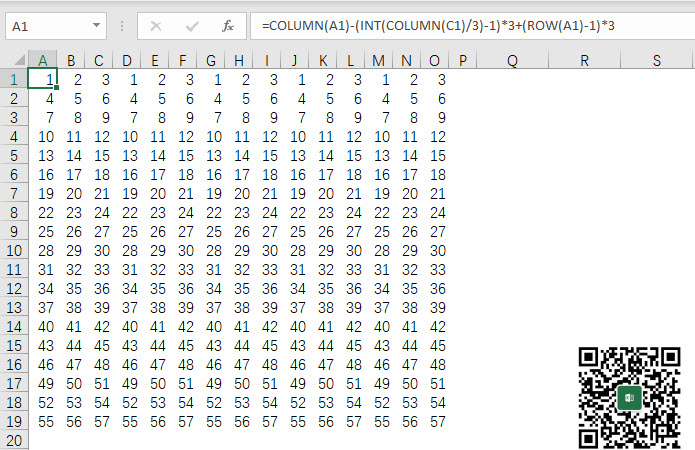﻿ 如何用函数公式生成123、123...这样重复的有规律的序号数列？ - Excel - ExcelOffice【微信公众号：水星Excel】

# 如何用函数公式生成123、123…这样重复的有规律的序号数列？`=COLUMN(A1)-(INT(COLUMN(C1)/3)-1)*3``=COLUMN(A1)-(INT(COLUMN(C1)/3)-1)*3+(ROW(A1)-1)*3`1.单个重复序号的数量，这里是123共计3个数，可以用M代替。

2.从左到右重复的次数，这里是3次，可以用N代替。

3.从上到下的等差数列的等差值，这里是3，可以用D代替。

`=COLUMN(A1)-(INT(COLUMN(C1)/3)-1)*3+(ROW(A1)-1)*3`

`=COLUMN(A1)-(INT(COLUMN(M所在的列)/M)-1)*N+(ROW(A1)-1)*D`